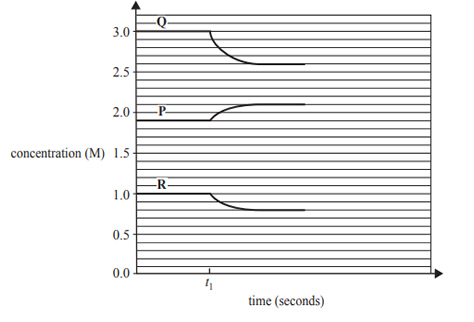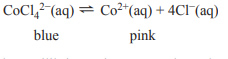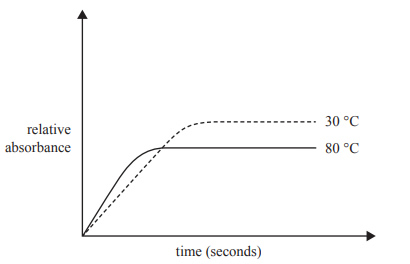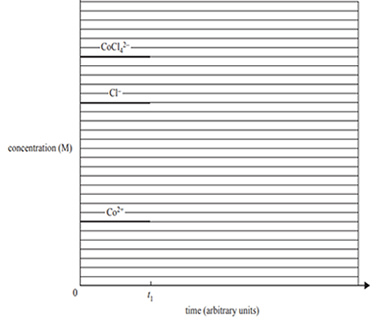Equilibrium (2019 VCE) 1) An understanding of Le Chatelier’s principle is useful in the chemical industry. The prediction that can be made using this principle is the effect of A. catalysts on the rate of reaction. B. catalysts on the position of equilibrium. C. changes in temperature on the rate of reaction. D. changes in the concentration of reactants on the position of equilibrium.. Solution2) The following concentration–time graph refers to a mixture of three gases, P, Q and R, in an enclosed 5.0 L container. At time t1 the mixture is heated.The equilibrium system that represents the graph is A. P(g) -> 2Q(g) + R(g) and the forward reaction is exothermic. B. 2Q(g) -> P(g) + R(g) and the forward reaction is endothermic. C. 2Q(g) + R(g) -> P(g) and the forward reaction is exothermic. D. P(g) + 2Q(g) -> R(g) and the forward reaction is endothermic. Solution3) The cobalt(II) tetrachloride ion, CoCl42−, dissociates into the cobalt(II) ion, Co2+, and chloride ions, Cl−, according to the following chemical equation.20 mL samples of the equilibrium mixture were heated to two temperatures, 30 °C and 80 °C. The intensity of the pink colour of the Co2+ product was recorded every 30 seconds by measuring the absorbance of the solution. The higher the intensity of the pink colour, the higher the absorbance. The results of this experiment are shown in the graph below.a) State whether the forward reaction is exothermic or endothermic. Justify your answer by referring to the graph. Solutionb) When 5 mL of water was added to the equilibrium mixture, the colour of the solution immediately became a lighter pink. Describe the final colour of the solution once equilibrium is re-established. Explain your answer Solutionc) Five drops of silver nitrate, AgNO3, solution are added to the equilibrium mixture at time t1. A concentration–time graph for this reaction is shown below for times between zero and t1. Continue the graph to show the changes that occur to the system from t1 until equilibrium is re-established.Solution